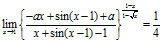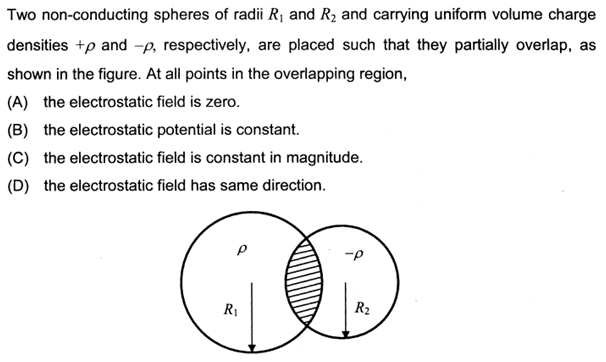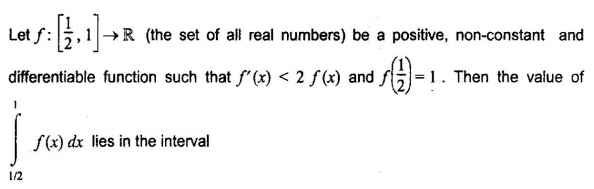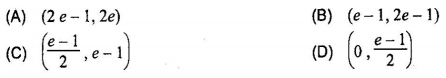# Largest Value of Non-Negative Integer $a$

The largest value of the nonnegative integer a for whichis

# JEE Advanced 2013 Physics Electrostatics Solution

More than one option may be correct.# Order of Reaction $P + Q \to R + S$

Problem

In the reaction,$\displaystyle P+Q\to R+S$

the time taken for 75% reaction of P is twice the time taken for 50% reaction of P. The concentration of Q varies with reaction time as shown in the figure. The overall order of the reaction is

(A) 2                                                         (B) 3
(C) 0                                                         (D) 1
Continue reading Order of Reaction $P + Q \to R + S$

# $\int\limits_{1/2}^1 {f(x)dx}$ lies in the interval